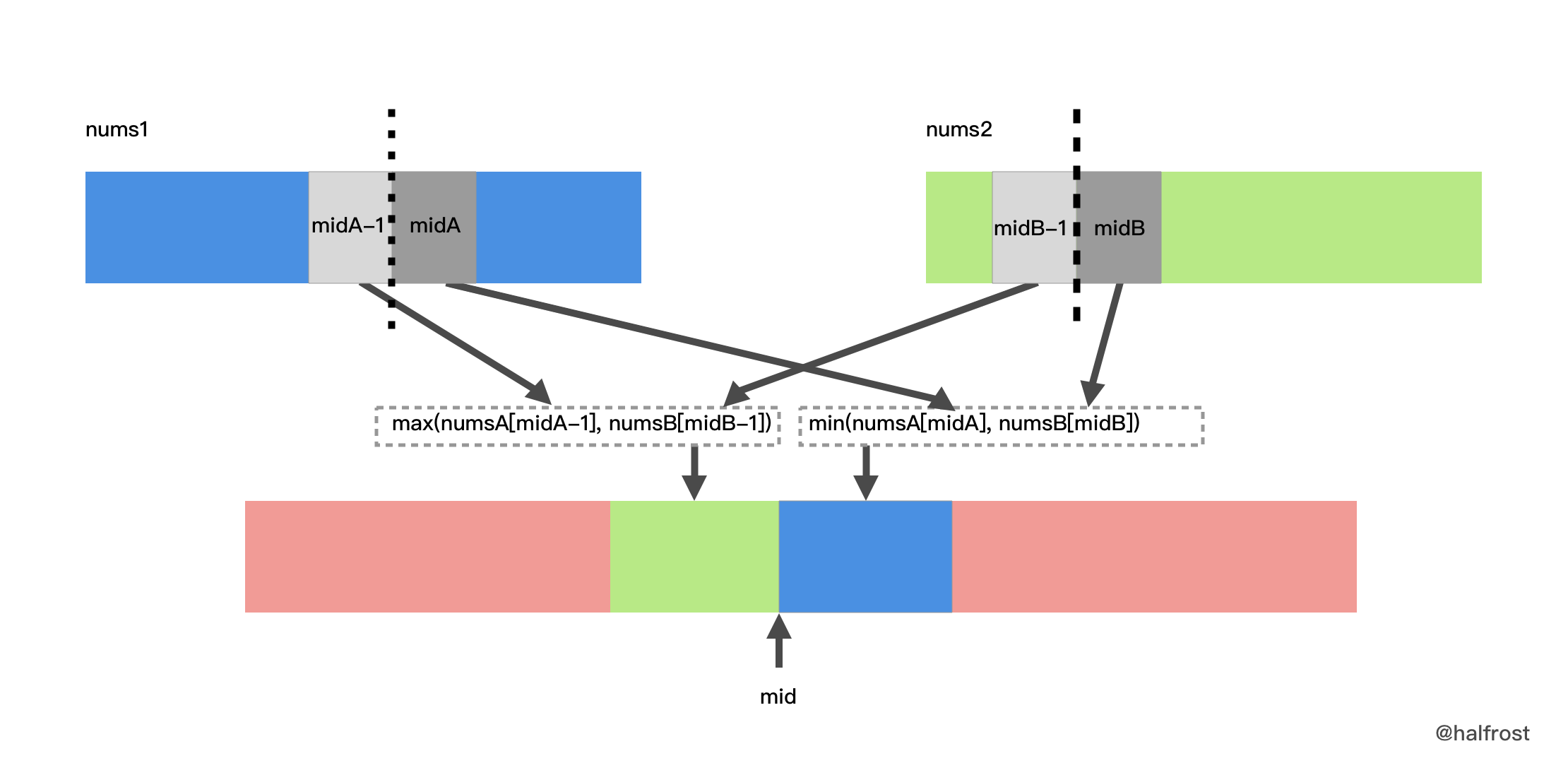## 每日一题

### 题目

There are two sorted arrays nums1 and nums2 of size m and n respectively.

Find the median of the two sorted arrays. The overall run time complexity should be O(log (m+n)).

You may assume nums1 and nums2 cannot be both empty.

Example 1:

nums1 = [1, 3]
nums2 = 

The median is 2.0

Example 2:

nums1 = [1, 2]
nums2 = [3, 4]

The median is (2 + 3)/2 = 2.5

### 结题思路

• 给出两个有序数组，要求找出这两个数组合并以后的有序数组中的中位数。要求时间复杂度为 O(log (m+n))。
• 这一题最容易想到的办法是把两个数组合并，然后取出中位数。但是合并有序数组的操作是 O(m+n) 的，不符合题意。看到题目给的 log 的时间复杂度，很容易联想到二分搜索。
• 由于要找到最终合并以后数组的中位数，两个数组的总大小也知道，所以中间这个位置也是知道的。只需要二分搜索一个数组中切分的位置，另一个数组中切分的位置也能得到。为了使得时间复杂度最小，所以二分搜索两个数组中长度较小的那个数组。
• 关键的问题是如何切分数组 1 和数组 2 。其实就是如何切分数组 1 。先随便二分产生一个 midA，切分的线何时算满足了中位数的条件呢？即，线左边的数都小于右边的数，即，nums1[midA-1] ≤ nums2[midB] && nums2[midB-1] ≤ nums1[midA] 。如果这些条件都不满足，切分线就需要调整。如果 nums1[midA] < nums2[midB-1]，说明 midA 这条线划分出来左边的数小了，切分线应该右移；如果 nums1[midA-1] > nums2[midB]，说明 midA 这条线划分出来左边的数大了，切分线应该左移。经过多次调整以后，切分线总能找到满足条件的解。
• 假设现在找到了切分的两条线了，数组 1 在切分线两边的下标分别是 midA - 1midA数组 2 在切分线两边的下标分别是 midB - 1midB。最终合并成最终数组，如果数组长度是奇数，那么中位数就是 max(nums1[midA-1], nums2[midB-1])。如果数组长度是偶数，那么中间位置的两个数依次是：max(nums1[midA-1], nums2[midB-1])min(nums1[midA], nums2[midB])，那么中位数就是 (max(nums1[midA-1], nums2[midB-1]) + min(nums1[midA], nums2[midB])) / 2。图示见下图：### 代码实现与注释

func findMedianSortedArrays(nums1 []int, nums2 []int) float64 {
// 假设 nums1 的长度小
if len(nums1) > len(nums2) {
//递归替换参数
return findMedianSortedArrays(nums2, nums1)
}
//初始化参数
low, high, k, nums1Mid, nums2Mid := 0, len(nums1), (len(nums1)+len(nums2)+1)>>1, 0, 0
for low <= high {
// nums1:  ……………… nums1[nums1Mid-1] | nums1[nums1Mid] ……………………
// nums2:  ……………… nums2[nums2Mid-1] | nums2[nums2Mid] ……………………
nums1Mid = low + (high-low)>>1 // 分界限右侧是 mid，分界线左侧是 mid - 1
nums2Mid = k - nums1Mid
if nums1Mid > 0 && nums1[nums1Mid-1] > nums2[nums2Mid] { // nums1 中的分界线划多了，要向左边移动
high = nums1Mid - 1
} else if nums1Mid != len(nums1) && nums1[nums1Mid] < nums2[nums2Mid-1] { // nums1 中的分界线划少了，要向右边移动
low = nums1Mid + 1
} else {
// 找到合适的划分了，需要输出最终结果了
// 分为奇数偶数 2 种情况
break
}
}
midLeft, midRight := 0, 0
if nums1Mid == 0 {
midLeft = nums2[nums2Mid-1]
} else if nums2Mid == 0 {
midLeft = nums1[nums1Mid-1]
} else {
midLeft = max(nums1[nums1Mid-1], nums2[nums2Mid-1])
}
if (len(nums1)+len(nums2))&1 == 1 {
return float64(midLeft)
}
if nums1Mid == len(nums1) {
midRight = nums2[nums2Mid]
} else if nums2Mid == len(nums2) {
midRight = nums1[nums1Mid]
} else {
midRight = min(nums1[nums1Mid], nums2[nums2Mid])
}
return float64(midLeft+midRight) / 2
}